# Correlation matrix

(diff) ← Older revision | Latest revision (diff) | Newer revision → (diff)
The matrix of correlation coefficients of several random variables. Ifare random variables with non-zero variances, then the matrix entries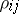() are equal to the correlation coefficients (cf. Correlation coefficient)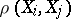; for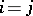the element is defined to be 1. The properties of the correlation matrix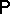are determined by the properties of the covariance matrix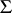, by virtue of the relation, whereis the diagonal matrix with (diagonal) entries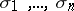.